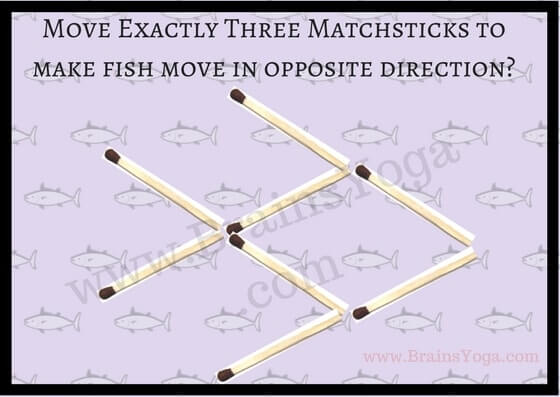There are many different types of picture puzzles. Matchsticks puzzles are one of the picture puzzle types. Some of the Matchsticks puzzles require Mathematical calculations. However, today's Matchstick puzzle can be solved without Maths skills. Can you solve this Matchstick puzzle?
Move exactly three matchsticks to make the fish move in the opposite direction.Picture Puzzle using matchsticks